## Black Box Paths

Blacken exactly 10 of the empty squares in each 7x7 grid (exactly 6 squares in each 5x5 grid), such that each numbered square indicates how many adjacent squares are black, looking horizontally, vertically or diagonally. In addition, all of the remaining squares not used by numbers or black squares can be connected by a single, closed loop (connecting squares horizontally or vertically, entering and exiting each empty square once.)

5x5 Puzzles. Blacken exactly 6 squares in each grid. I made the 7x7 puzzles first, but I thought some 5x5 puzzles would be good for warmups.
 ```A.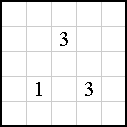``` ` ` ```B.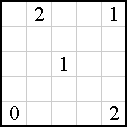``` ` ` ```C.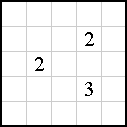```
7x7 Puzzles. Blacken exactly 10 squares in each grid. Puzzle A was the first of these puzzles I created. In B, I started trying to put only 2s in the diagram, but ended with this. In C, I wanted to put the numbers 1, 2, 3 and 4 in the four corners. In D, I was aiming for a nice arrangement of the numbers; the black squares and path may or may not be as nicely arranged in the solution.
 ```A. Puzzle One (first made)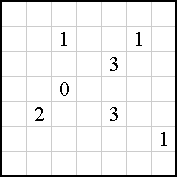``` ` ` ```B. Mostly 2s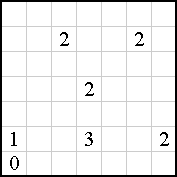``` ```C. 1234 Corners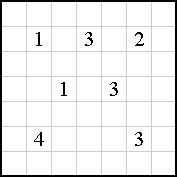``` ` ` ```D. The Flower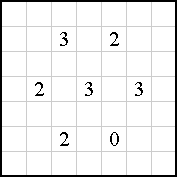```
Update 07/26/02: More 7x7 Puzzles. Blacken exactly 10 squares in each grid. I found I could place only five numbers as clues and still create a puzzle with a unique solution.
 ```E. Ones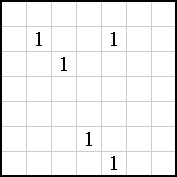``` ` ` ```F. Twos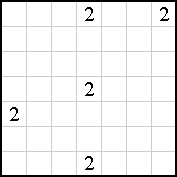``` ```G. Threes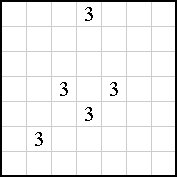``` ` ` ```H. Fours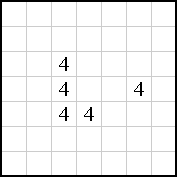```
For sending solutions, unless you're going to send a graphical solution I can read and post, you're welcome to just send an "I solved it" with any comments you might have. I believe each of the puzzles above has a unique solution (creating them that way took quite a while), but perhaps someone will prove me wrong.

Source: Original. Created by combining features of both the "Black Magic" puzzles from Dell Math Puzzles and Logic Problems magazine and question 22 "Path Battleships" of the 2002 WPC Qualifying Test (http://wpc.puzzles.com). My thanks to Cihan Altay for making the images for me.

Solution
Mail to Ken